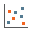Join one of our upcoming courses taking place around the world! Explore now

# Scatter Plot

A Scatter Plot in Slide2 allows you to plot any two random variables from a Probabilistic Analysis, against each other on the same plot. Typically, the Scatter Plot is used to plot Factor of Safety versus an input data Random Variable, to observe the correlation between the two.

To create a Scatter Plot:

1. Select Scatter Plotfrom the toolbar or the Statistics menu.
2. In the Scatter Plot dialog, select the data you would like to plot, on the Horizontal Axis and the Vertical Axis. The following data can be selected, for either axis:
• Any Random Variable that you have defined for the Probabilistic Analysis.
• The Factor of Safety for any analysis method which was used.
3. You can choose to highlight data on the plot, according to user-defined criteria, by selecting the Highlight Data checkbox, and defining your criteria. See below for details.
4. Select the Plot button to generate the Scatter Plot.
5. Once a plot is generated, there are many different options available, if you right-click the mouse on the plot. See below for details.

## Highlight Data

The Highlight Data option for Scatter Plots works in the same manner as described for a Histogram Plot. See the Histogram Plot topic for details. On a Scatter Plot, when data is highlighted, the data points will be displayed as RED SQUARES on the plot.

## Correlation Coefficient

The Correlation Coefficient indicates the degree of correlation between the two variables plotted. The Correlation Coefficient can vary between -1 and 1 where numbers close to zero indicate a poor correlation, and numbers close to 1 or -1 indicate a good correlation. Note that a negative correlation coefficient simply means that the slope of the best fit linear regression line is negative.

## User-Defined Correlation Coefficient

In the Slide2 Model program, you can specify a user-defined correlation coefficient, between Cohesion and Friction Angle, for a Mohr-Coulomb material. In this case, the two variables will automatically be correlated, when the samples are created. You can use a Scatter Plot to observe the actual correlation between the two variables, which was generated by the sampling. The actual correlation should be close (but not necessarily equal) to the value you have specified. However, this may not always be true, especially if you have specified a correlation coefficient close to 1 (or -1), and you are using distributions other than the Normal Distribution.

## Alpha and Beta

Alpha and beta represent the y-intercept and slope, respectively, of the best fit linear regression line to the scatter plot data. The display of the linear regression line can be toggled on or off, by selecting the Regression Line option in the right-click menu, on a Scatter Plot.

## Right-Click Options

Other options are available if you right-click on a Scatter Plot. For example:

• Select the Change Plot Data option to change the data viewed on the plot.
• Select the Chart Properties option to customize the appearance of the plot.
• The Sampler option allows you to graphically determine the coordinates of any data point on a Scatter Plot. For more information, see the discussion of the Sampler for the Cumulative Plot, as the Scatter Plot Sampler operates in a similar manner.

Experiment with the different options available in the right-click menu.